# Consider the beam shown in (Figure 1). EI is constant. Assume A is a pin. Suppose...

Consider the beam shown in (Figure 1). EI is constant. Assume A is a pin. Suppose that w = 1.4 k/ft, and EI is in k?ft2.

Determine the slope at point C measured counterclockwise from the positive x axis. Use the method of virtual work.

Determine the displacement at point C measured downward. Use the method of virtual work.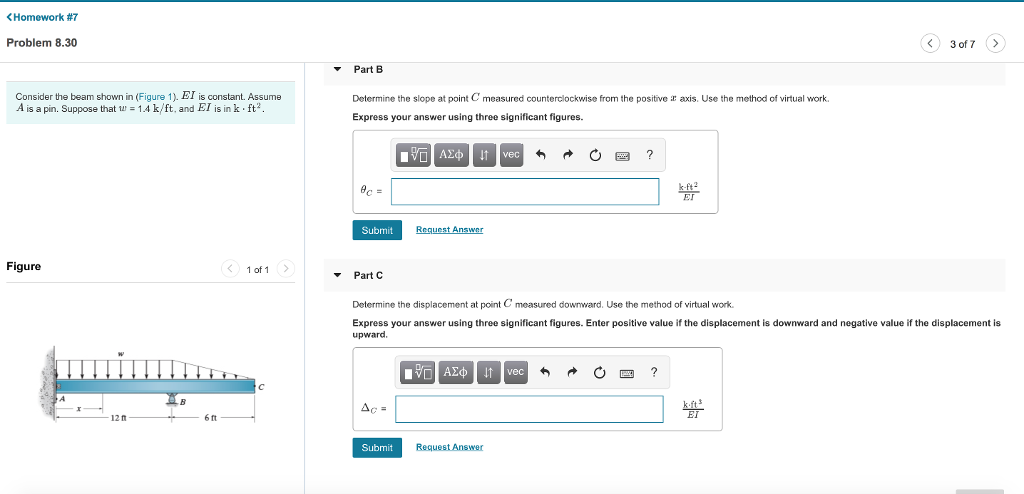Please use the method of virtual work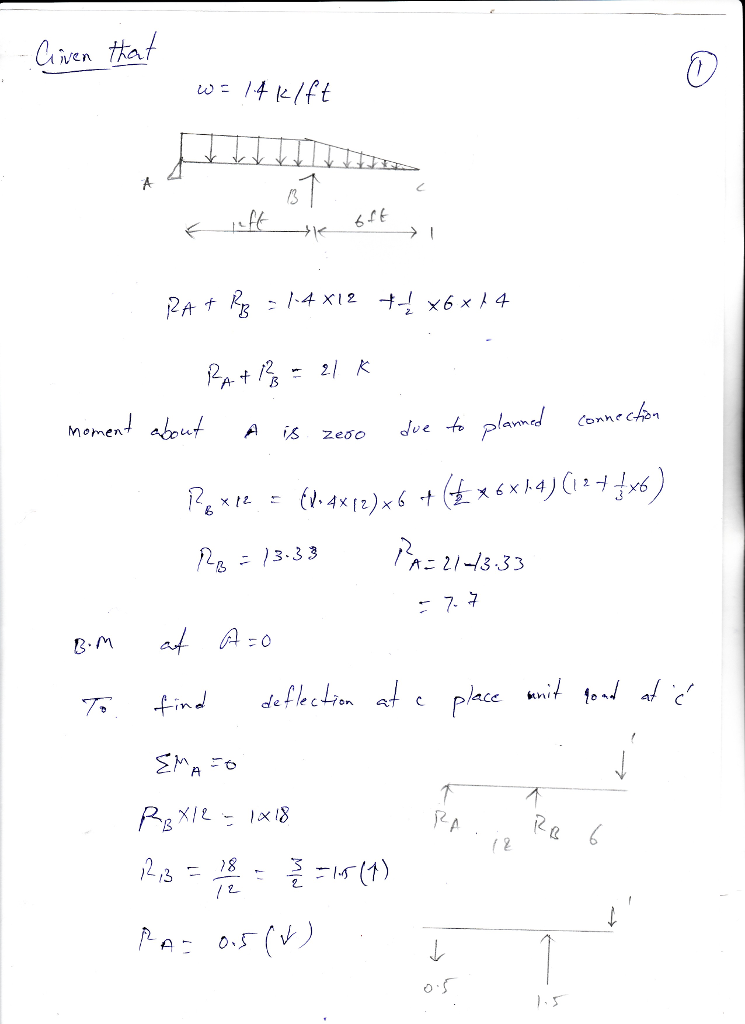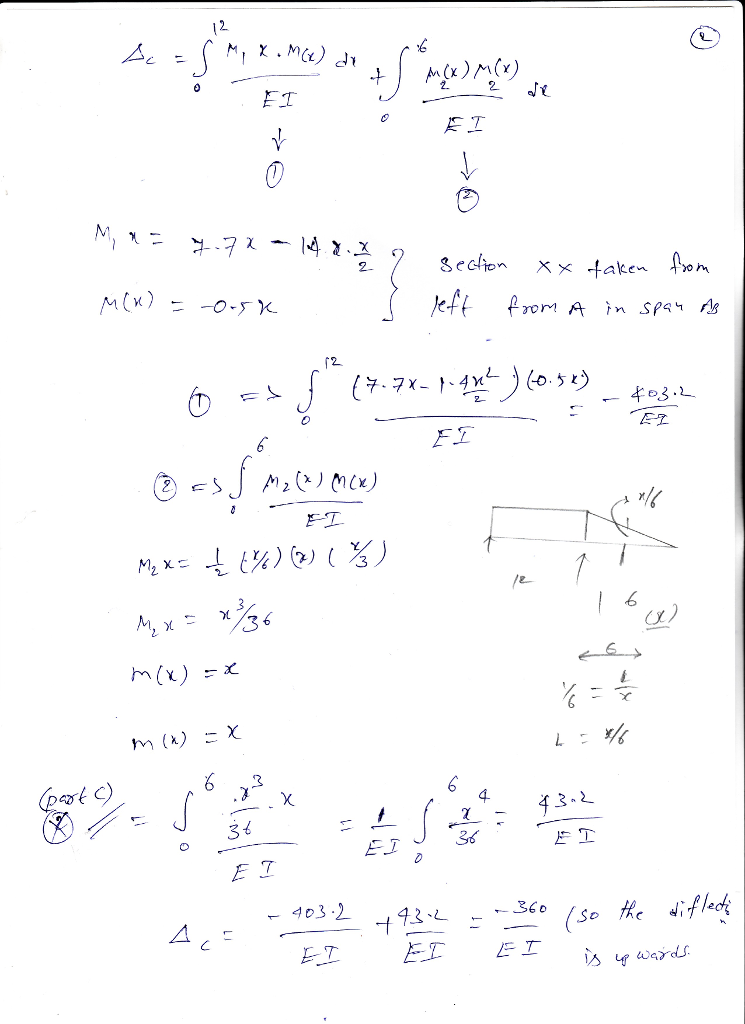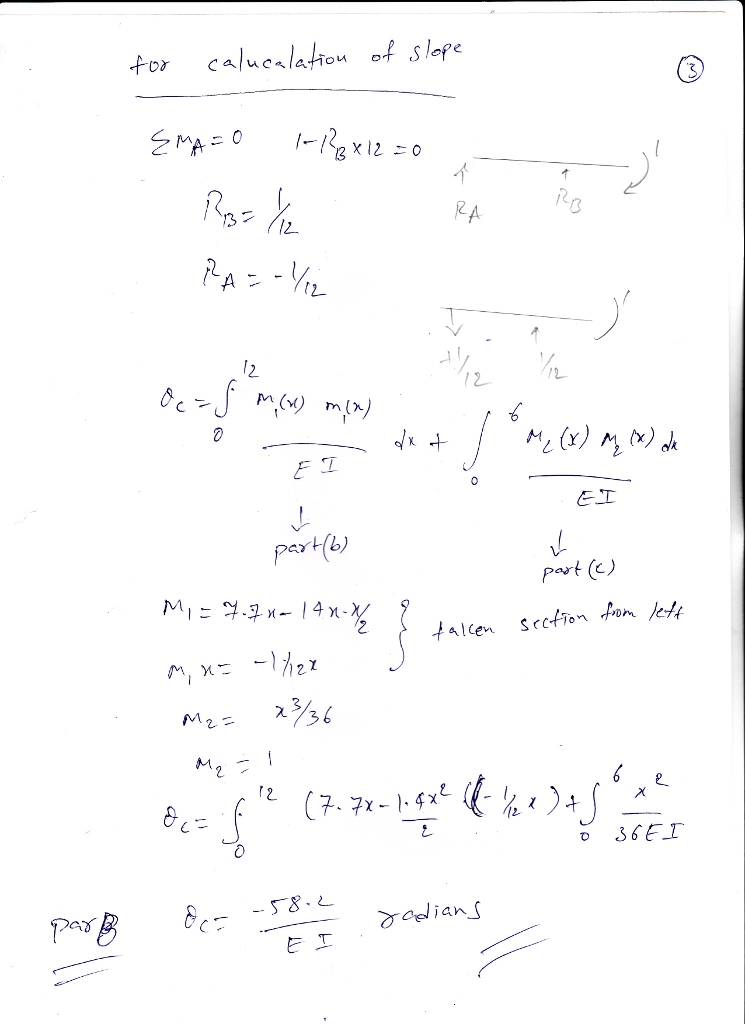##### Add Answer to: Consider the beam shown in (Figure 1). EI is constant. Assume A is a pin. Suppose...
Similar Homework Help Questions
• ### Consider the beam shown in (Figure 1). El is constant. Use the conjugate beam method. Suppose...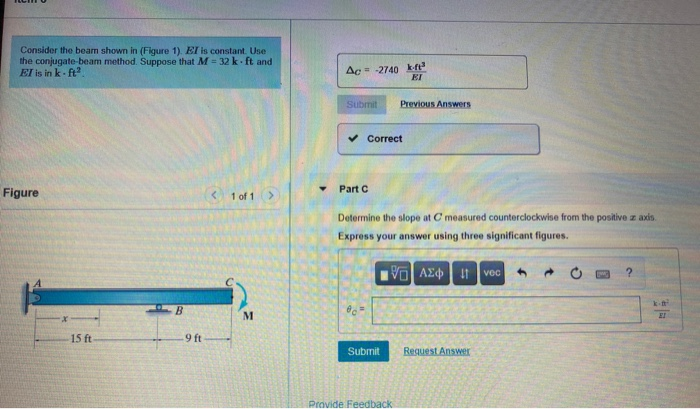Consider the beam shown in (Figure 1). El is constant. Use the conjugate beam method. Suppose that M = 32 kft and EI is in kft Ac = -2740 kr ET Submit Previous Answers Correct Part Figure 1 of 1 > Determine the slope at C measured counterclockwise from the positive z axis Express your answer using three significant figures. VE ΑΣΦ 11 VOC h ? B M 15 ft 9 ft Submit Request Answer Pravide Feedback

• ### Consider the beam shown in (Figure 1). EI is constant. Assume B and C are rollers...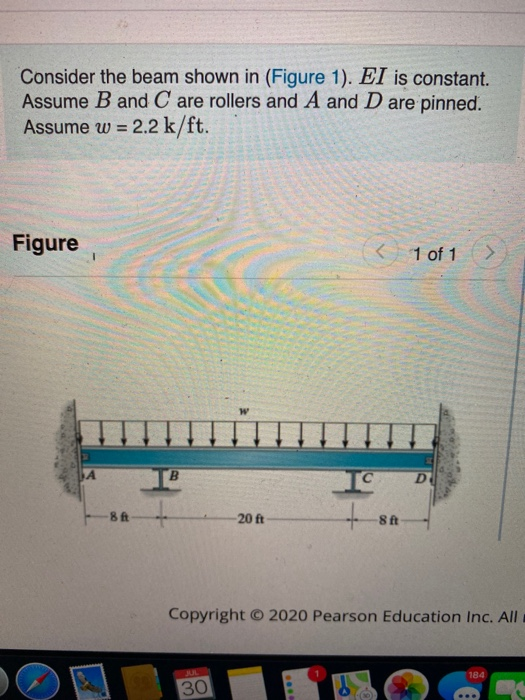Consider the beam shown in (Figure 1). EI is constant. Assume B and C are rollers and A and D are pinned. Assume w = 2.2 k/ft. Figure < 1 of 1 > w IA D 8 ft -20 ft 8 ft Copyright © 2020 Pearson Education Inc. All 184 30 3 of 3 Part A Determine the moment MBA acting on span AB at B measured clockwise. Express your answer using three significant figures. Enter positive value if the...

• ### Consider the beam shown in (Figure 1). EI is constant. Assume B and C are rollers...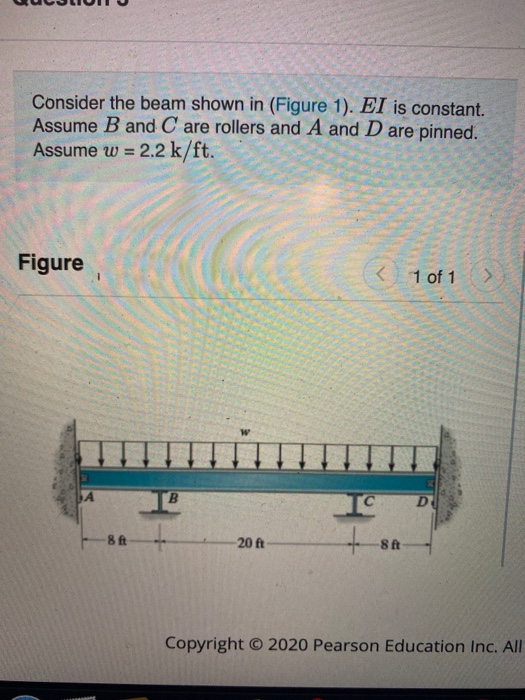Consider the beam shown in (Figure 1). EI is constant. Assume B and C are rollers and A and D are pinned. Assume w = 2.2 k/ft. Figure < - 1 of 1 > IA D 8 ft -20 ft 8 ft Copyright © 2020 Pearson Education Inc. All 3 of 3 Part A Determine the moment MBA acting on span AB at B measured clockwise. Express your answer using three significant figures. Enter positive value if the moment is...

• ### Part A Consider the truss shown in (Figure 1). Assume the members are pin connected at...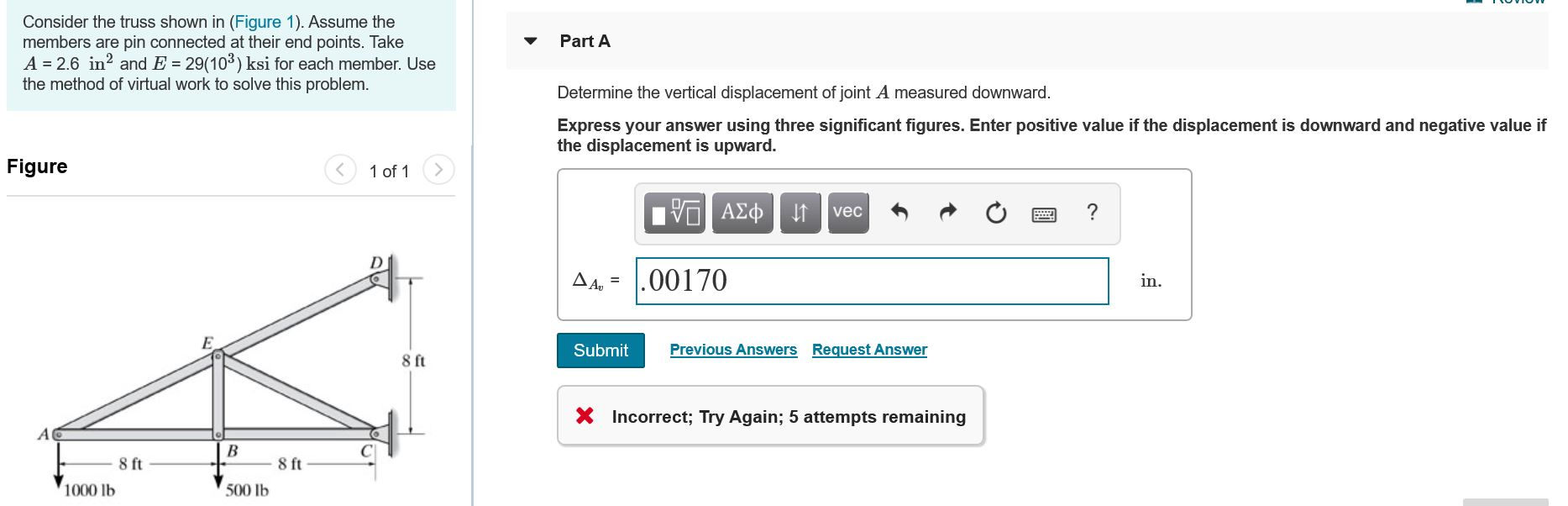Part A Consider the truss shown in (Figure 1). Assume the members are pin connected at their end points. Take A = 2.6 in? and E = 29(103) ksi for each member. Use the method of virtual work to solve this problem. Determine the vertical displacement of joint A measured downward. Express your answer using three significant figures. Enter positive value if the displacement is downward and negative value if the displacement is upward. Figure < 1 of 1 V...

• ### Determine the slope and displacement at point C. El is constant. Assume A is a pin....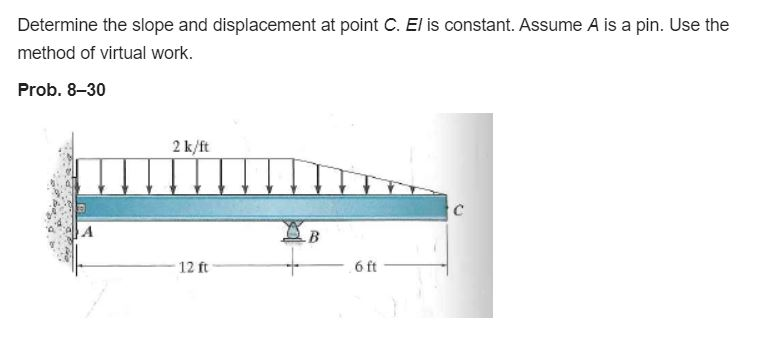Determine the slope and displacement at point C. El is constant. Assume A is a pin. Use the method of virtual work. Prob. 8-30 2 k/ft с А 12 ft 6 ft

• ### Consider the beam shown in (Figure 1). Suppose that M = 75 kft. Er is constant....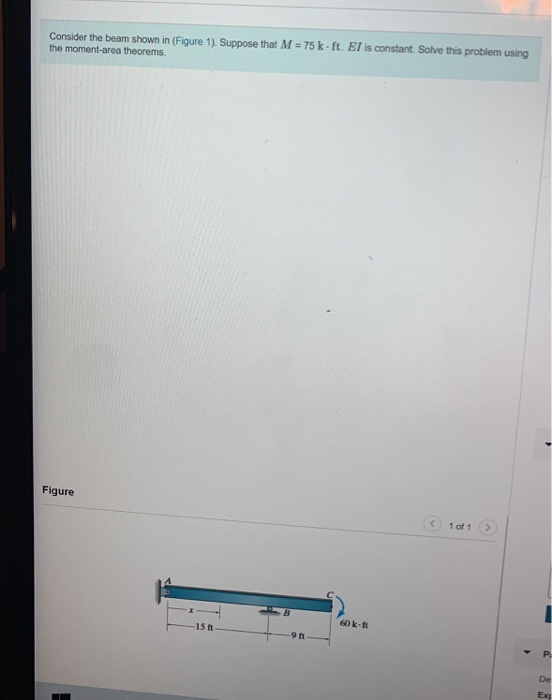Consider the beam shown in (Figure 1). Suppose that M = 75 kft. Er is constant. Solve this problem using the moment-area theorems. Figure 1 of 1 -15 ft 60k- 9 ft P De the angles and deviations betwo Part B Determine the displacement at C measured upward. Express your answer using three significant figures. View Available Hint(s) I AEP ! vec Ik-ft Submit Part 1 of 1 Determine the slope at C measured counterclockwise from the positive r axis....

• ### Consider the beam shown in (Figure 1) E - 29(10) ksi and I 202 in Part...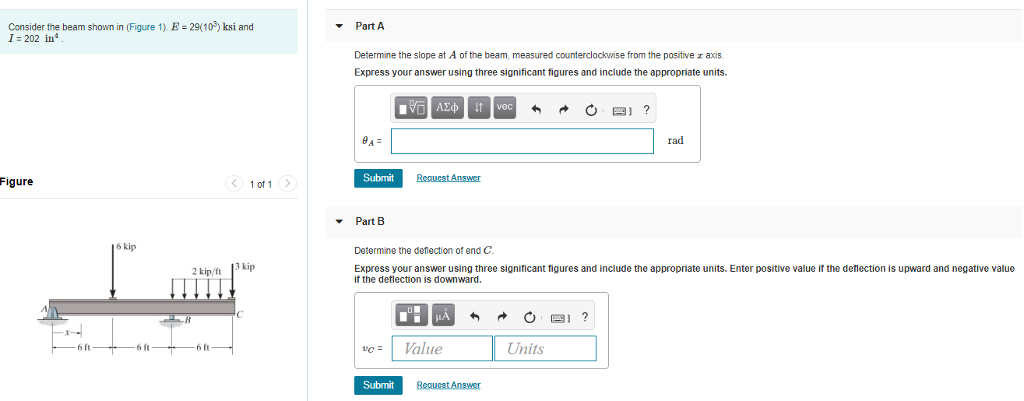Consider the beam shown in (Figure 1) E - 29(10) ksi and I 202 in Part A Determine the slope at A of the beam, measured counterclockwise from the positive r axis Express your answer using three significant figures and include the appropriate units. rad Submit Figure Part B Determine the defection of end C Express your answer using three significant tigures and include the appropriate units. Enter positive value if the deflection is upward and negative value if the...

• ### Consider the frame shown in (Figure 1). Assume A and D are pins. EI is constant. Suppose that w- 2 k/ft. 9 k 12 ft 8...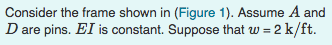Consider the frame shown in (Figure 1). Assume A and D are pins. EI is constant. Suppose that w- 2 k/ft. 9 k 12 ft 8 ft Determine the component of reaction at D Consider the frame shown in (Figure 1). Assume A and D are pins. EI is constant. Suppose that w- 2 k/ft. 9 k 12 ft 8 ft Determine the component of reaction at D

• ### Question 1 < 1 of 3 Consider the beam shown in (Figure 1). Assume A is...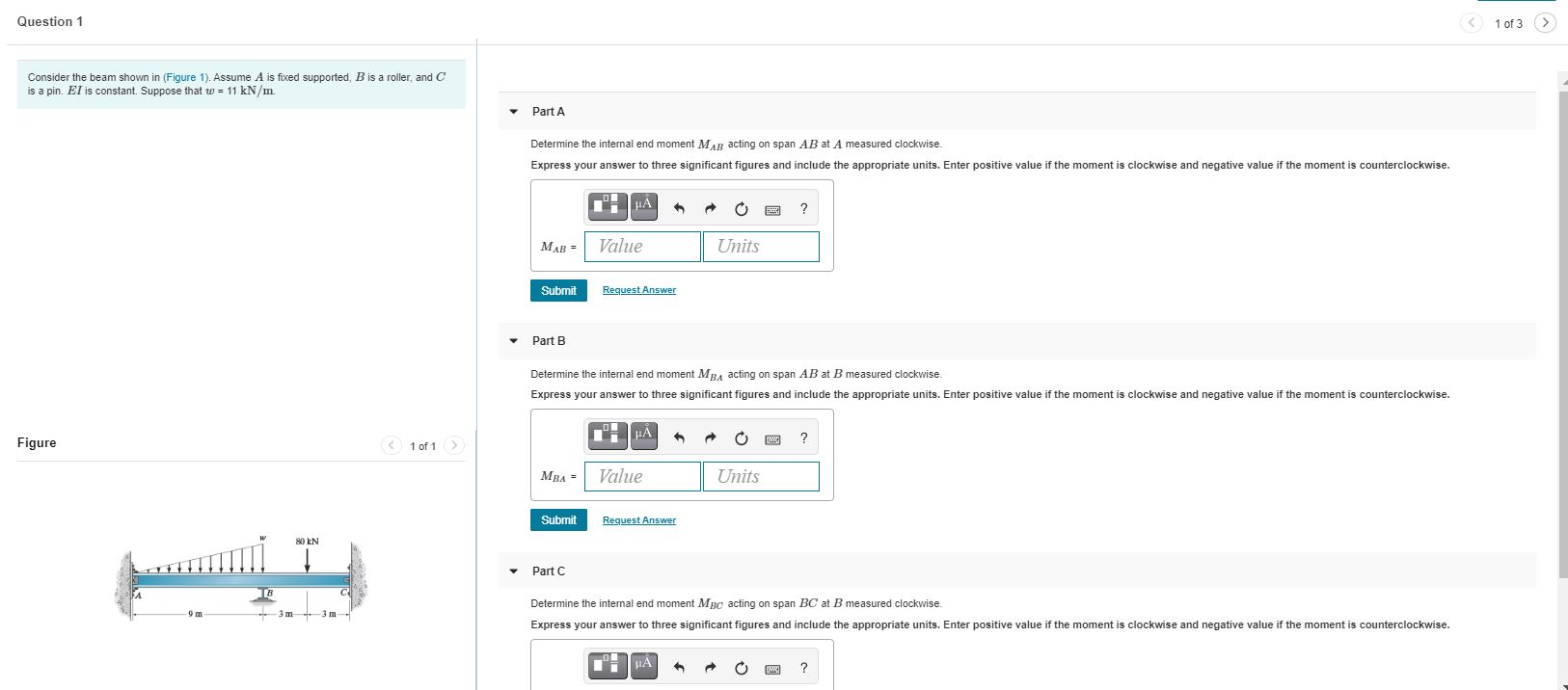Question 1 < 1 of 3 Consider the beam shown in (Figure 1). Assume A is fixed supported, B is a roller, and C is a pin. Er is constant. Suppose that w = 11 kN/m. Part A Determine the internal end moment MAB acting on span AB at measured clockwise. Express your answer to three significant figures and include the appropriate units. Enter positive value if the moment is clockwise and negative value if the moment is counterclockwise. μΑ...

• ### Question 1 < 1 of 3 Consider the beam shown in (Figure 1). Assume A is...Question 1 < 1 of 3 Consider the beam shown in (Figure 1). Assume A is fixed supported, B is a roller, and C is a pin. Er is constant. Suppose that w = 11 kN/m. Part A Determine the internal end moment MAB acting on span AB at measured clockwise. Express your answer to three significant figures and include the appropriate units. Enter positive value if the moment is clockwise and negative value if the moment is counterclockwise. μΑ...

Free Homework App